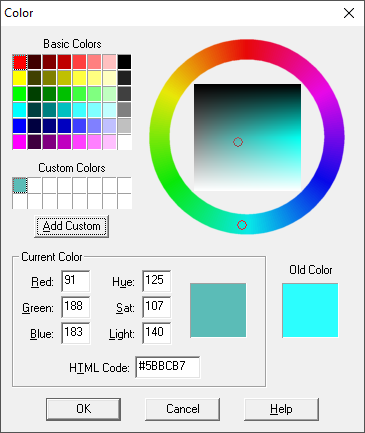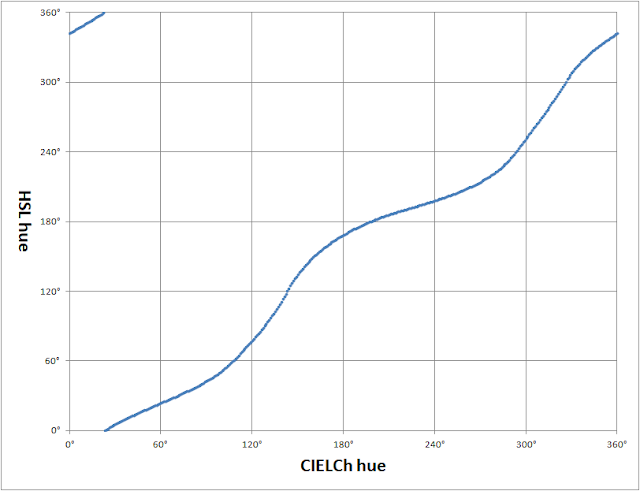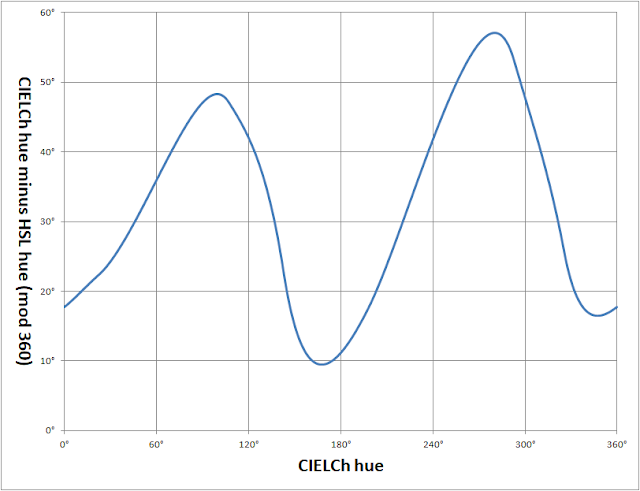## Tuesday, 23 March 2021

### HSL and CIELCh(ab) Hue Wheels

I've been looking at colour pickers recently and came across (what I think is) an interesting cul-de-sac.

HSL colour pickers often have a hue wheel similar to this one from Paint Shop Pro 5 (1998):Paint Shop Pro 5 colour picker dialog

The wheel is rendered as an angle sweeps from 0 to 360 degrees clockwise:

output = HSL_to_RGB(angle, 100%, 50%)

The HSL wheel looks like this:HSL hue wheel

Note that the "hue" components of HSL, HSV and HWB are identical. However, this is not the case for the cylindrical version of CIE Lab, a.k.a. CIELCh(ab).

We can construct the CIELCh(ab) hue wheel as follows:

rgb = CIELChab_to_sRGB(70, 30, angle)
hsl = RGB_to_HSL(rgb)
output = HSL_to_RGB(hsl.h, 100%, 50%)

We choose a CIE perceptual lightness (L*) of 70 and chroma (C*) of 30 so as not to go out-of-gamut when converting to sRGB. We also implicitly assume the reference white to be the CIE standard illuminant D65 and a 2-degree observer angle.

We use HSL to fully saturate the output colour to produce the following wheel:CIELCh(ab) hue wheel

Note that the colours have shifted somewhat. This becomes clear if we plot both wheels on the same chart:HSL (outer) and CIELCh(ab) (inner) hue wheels

There's already an excellent comparison of these two wheels by Elle Stone, so I won't go into that here.

For reasons that will hopefully become clear in a later post, what I really wanted to do was plot the CIELCh(ab) hue wheel efficiently in WebGL. Who wouldn't?

I coded "CIELChab_to_sRGB" et al in GLSL (more on that later too) but felt a bit queasy performing such complicated arithmetic, particularly when the results get shunted through an RGB-HSL-RGB hack at the end. There must be an efficient way of translating CIELCh(ab) hue to HSL hue, surely?

If we plot the relationship, we get:CIELCh(ab) hue versus HSL hue

I looks like a fairly linear albeit wavy relationship, doesn't it? So let's plot the difference modulo 360 degrees:Difference in hues modulo 360 degrees

It now looks fairly sinusoidal, so maybe we can approximate it with the sum of a few sine waves.

If the input is the CIELCh(ab) hue angle in degrees and the desired output is the HSL hue, we can write:

output = input + c + a0*sin(b0+input) + a1*sin(b1+2*input) + a2*sin(b2+3*input) + …

It turns out you only need four sine terms to get a good approximation and Microsoft Excel Solver is more that capable of finding appropriate values for the offset "c", scale factors "a0" to "a3" and phase shifts "b0" to "b3".

I initially coded the formula as a GLSL function that takes the hues in the range zero to one, like so:

float hstar2hue(float input) {
// Convert CLIELCh(ab) hue [0..1] to HSV/HSL/HWB hue [0..1]
float a0 = 0.011681489;
float a1 = -0.053365543;
float a2 = -0.00331377;
float a3 = -0.009398634;
float b0 = -0.527823872;
float b1 = -1.606202694;
float b2 = 1.054897946;
float b3 = 0.102411421;
float c = 0.91047088;
float output = input + c;
output += a0 * sin(b0 + input * 2.0 * 3.1415926536);
output += a1 * sin(b1 + input * 4.0 * 3.1415926536);
output += a2 * sin(b2 + input * 6.0 * 3.1415926536);
output += a3 * sin(b3 + input * 8.0 * 3.1415926536);
return mod(output, 1.0);
}

But we can take advantage of GLSL's SIMD nature and get:

float hstar2hue(float input) {
// Convert CLIELCh(ab) hue [0..1] to HSV/HSL/HWB hue [0..1]
vec4 a = vec4(0.011681489, -0.053365543, -0.00331377, -0.009398634);
vec4 b = vec4(-0.527823872, -1.606202694, 1.054897946, 0.102411421);
float c = 0.91047088;
vec4 v = sin(vec4(1.0, 2.0, 3.0, 4.0) * input * 6.28318530718 + b);
return mod(input + dot(a, v) + c, 1.0);
}

It's then just a question of converting the HSL-style hue to RGB:

vec3 hue2rgb(float h) {
float r = abs(h * 6.0 - 3.0) - 1.0;
float g = 2.0 - abs(h * 6.0 - 2.0);
float b = 2.0 - abs(h * 6.0 - 4.0);
return clamp(vec3(r, g, b), 0.0, 1.0);
}

And we've got all the ingredients for a fragment shader to render the CIELCh(ab) hue wheel.

I'll leave it as an exercise for the reader to work out the reverse HSL-to-CIELCh(ab) hue approximation as well as those for CIELCh(uv).